# Deep attractors: Where deep learning meets chaos

In nonlinear dynamics, when the state space is thought to be multidimensional but all we have for data is just a univariate time series, one may attempt to reconstruct the true space via delay coordinate embeddings. However, it is not clear a priori how to choose dimensionality and time lag of the reconstruction space. In this post, we show how to use an autoencoder architecture to circumvent the problem: Given just a scalar series of observations, the autoencoder directly learns to represent attractors of chaotic systems in adequate dimensionality.

Sigrid Keydana (RStudio)https://www.rstudio.com/
06-24-2020

For us deep learning practitioners, the world is – not flat, but – linear, mostly. Or piecewise linear.1 Like other linear approximations, or maybe even more so, deep learning can be incredibly successful at making predictions. But let’s admit it – sometimes we just miss the thrill of the nonlinear, of good, old, deterministic-yet-unpredictable chaos. Can we have both? It looks like we can. In this post, we’ll see an application of deep learning (DL) to nonlinear time series prediction – or rather, the essential step that predates it: reconstructing the attractor underlying its dynamics. While this post is an introduction, presenting the topic from scratch, further posts will build on this and extrapolate to observational datasets.

### What to expect from this post

In his 2020 paper Deep reconstruction of strange attractors from time series , William Gilpin uses an autoencoder architecture, combined with a regularizer implementing the false nearest neighbors statistic , to reconstruct attractors from univariate observations of multivariate, nonlinear dynamical systems. If you feel you completely understand the sentence you just read, you may as well directly jump to the paper – come back for the code though2. If, on the other hand, you’re more familiar with the chaos on your desk (extrapolating … apologies) than chaos theory chaos, read on. Here, we’ll first go into what it’s all about3, and then, show an example application, featuring Edward Lorenz’s famous butterfly attractor. While this initial post is primarily supposed to be a fun introduction to a fascinating topic, we hope to follow up with applications to real-world datasets in the future.

## Rabbits, butterflies, and low-dimensional projections: Our problem statement in context

In curious misalignment with how we use “chaos” in day-to-day language, chaos, the technical concept, is very different from stochasticity, or randomness. Chaos may emerge from purely deterministic processes - very simplistic ones, even. Let’s see how; with rabbits.

### Rabbits, or: Sensitive dependence on initial conditions

You may be familiar with the logistic equation, used as a toy model for population growth. Often it’s written like this – with $$x$$ being the size of the population, expressed as a fraction of the maximal size (a fraction of possible rabbits, thus), and $$r$$ being the growth rate (the rate at which rabbits reproduce):

$x_{n + 1} = r \ x_n \ (1 - x_n)$

This equation describes an iterated map over discrete timesteps $$n$$. Its repeated application results in a trajectory describing how the population of rabbits evolves. Maps can have fixed points, states where further function application goes on producing the same result forever. Example-wise, say the growth rate amounts to $$2.1$$, and we start at two (pretty different!) initial values, $$0.3$$ and $$0.8$$. Both trajectories arrive at a fixed point – the same fixed point – in fewer than 10 iterations. Were we asked to predict the population size after a hundred iterations, we could make a very confident guess, whatever the of starting value. (If the initial value is $$0$$, we stay at $$0$$, but we can be pretty certain of that as well.)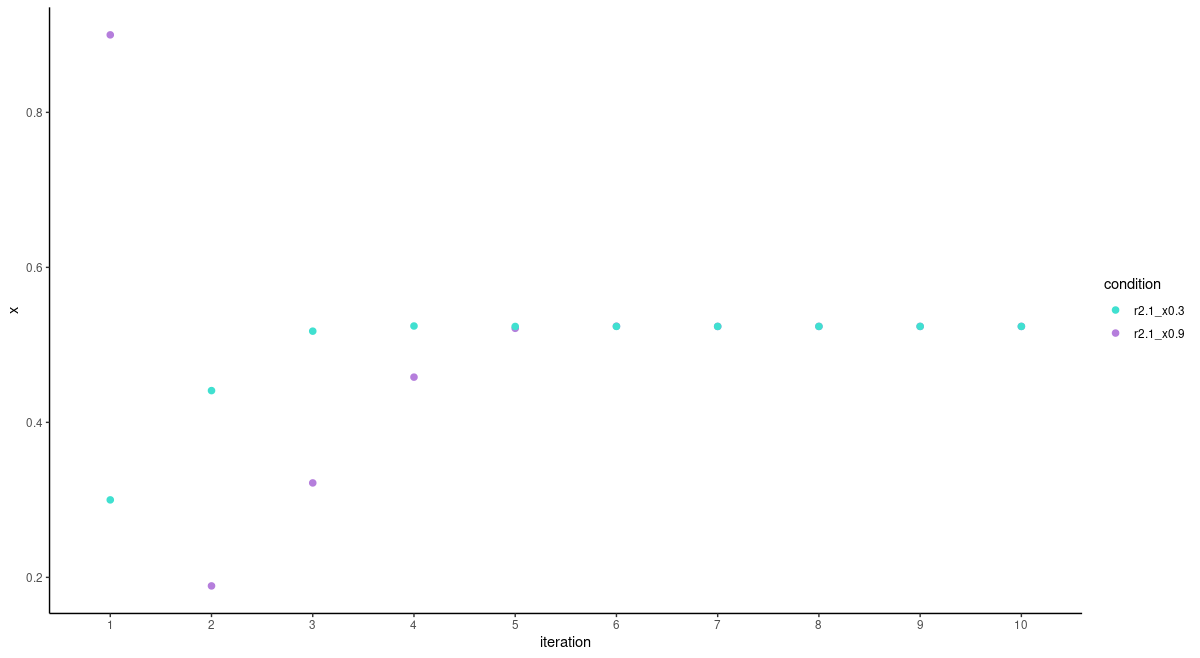Figure 1: Trajectory of the logistic map for r = 2.1 and two different initial values.

What if the growth rate were somewhat higher, at $$3.3$$, say? Again, we immediately compare trajectories resulting from initial values $$0.3$$ and $$0.9$$: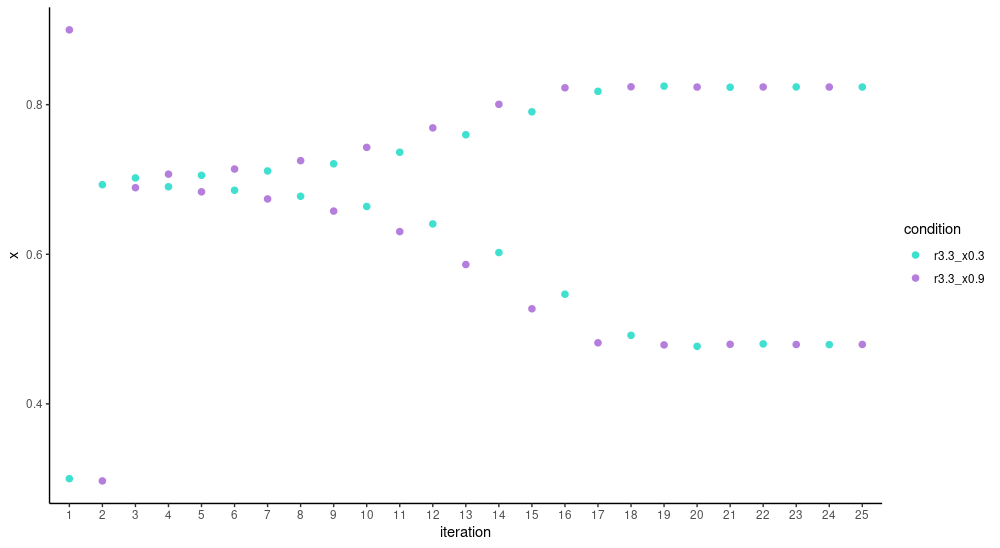Figure 2: Trajectory of the logistic map for r = 3.3 and two different initial values.

This time, don’t see a single fixed point, but a two-cycle: As the trajectories stabilize, population size inevitably is at one of two possible values – either too many rabbits or too few, you could say. The two trajectories are phase-shifted, but again, the attracting values – the attractor – is shared by both initial conditions. So still, predictability is pretty high. But we haven’t seen everything yet.

Let’s again enhance the growth rate some. Now this (literally) is chaos: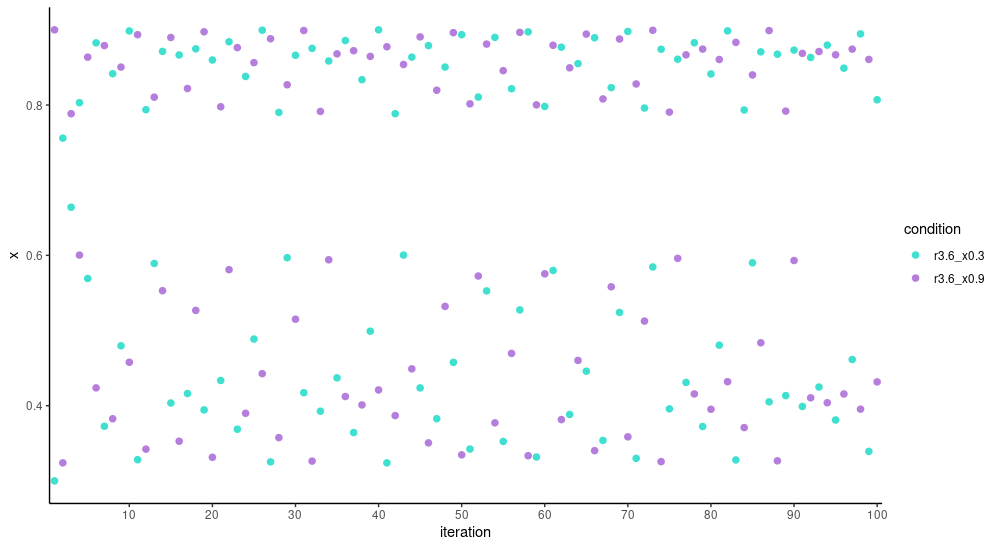Figure 3: Trajectory of the logistic map for r = 3.6 and two different initial values, 0.3 and 0.9.

Even after a hundred iterations, there is no set of values the trajectories recur to. We can’t be confident about any prediction we might make.

Or can we? After all, we have the governing equation, which is deterministic. So we should be able to calculate the size of the population at, say, time $$150$$? In principle, yes; but this presupposes we have an accurate measurement for the starting state.

How accurate? Let’s compare trajectories for initial values $$0.3$$ and $$0.301$$: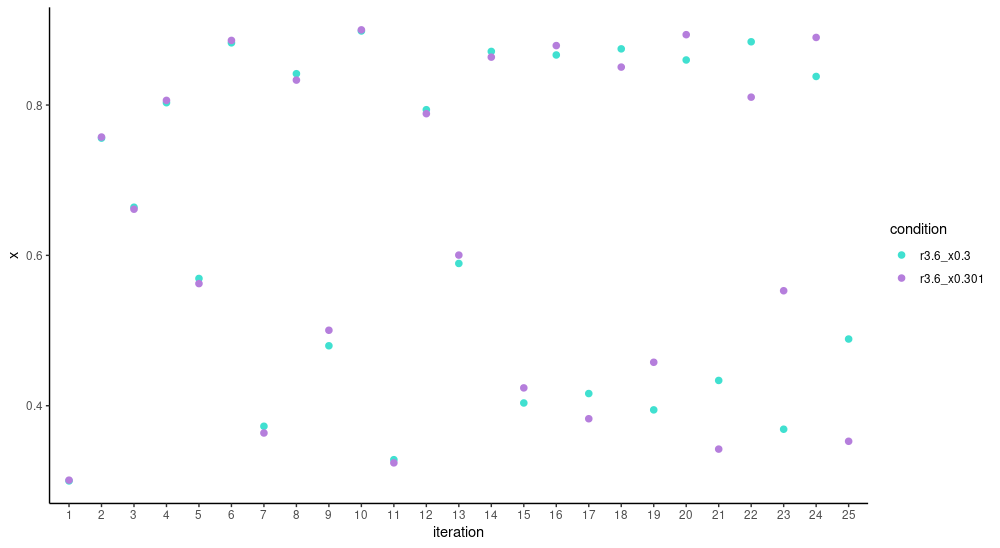Figure 4: Trajectory of the logistic map for r = 3.6 and two different initial values, 0.3 and 0.301.

At first, trajectories seem to jump around in unison; but during the second dozen iterations already, they dissociate more and more, and increasingly, all bets are off. What if initial values are really close, as in, $$0.3$$ vs. $$0.30000001$$?

It just takes a bit longer for the disassociation to surface.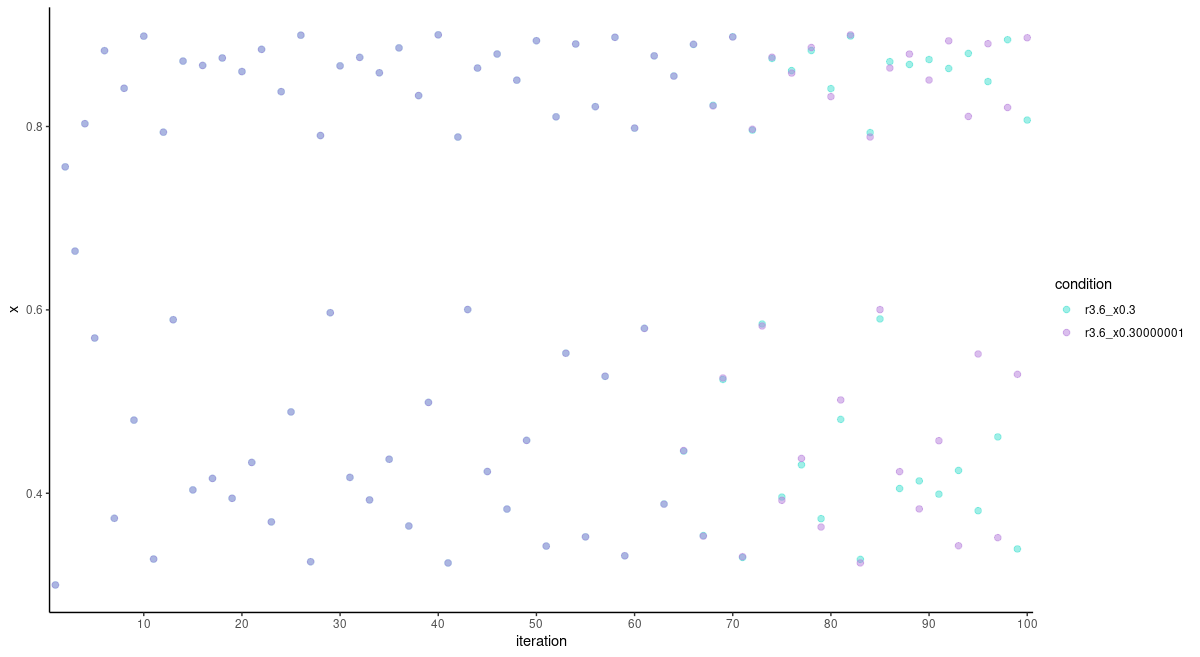Figure 5: Trajectory of the logistic map for r = 3.6 and two different initial values, 0.3 and 0.30000001.

What we’re seeing here is sensitive dependence on initial conditions, an essential precondition for a system to be chaotic. In an nutshell: Chaos arises when a deterministic system shows sensitive dependence on initial conditions. Or as Edward Lorenz is said to have put it,

When the present determines the future, but the approximate present does not approximately determine the future.

Now if these unstructured, random-looking point clouds constitute chaos, what with the all-but-amorphous butterfly (to be displayed very soon)?

### Butterflies, or: Attractors and strange attractors

Actually, in the context of chaos theory, the term butterfly may be encountered in different contexts.

Firstly, as so-called “butterfly effect,” it is an instantiation of the templatic phrase “the flap of a butterfly’s wing in _________ profoundly affects the course of the weather in _________.”4 In this usage, it is mostly a metaphor for sensitive dependence on initial conditions.

Secondly, the existence of this metaphor led to a Rorschach-test-like identification with two-dimensional visualizations of attractors of the Lorenz system. The Lorenz system is a set of three first-order differential equations designed to describe atmospheric convection:

\begin{aligned} & \frac{dx}{dt} = \sigma (y - x)\\ & \frac{dy}{dt} = \rho x - x z - y\\ & \frac{dz}{dt} = x y - \beta z \end{aligned}

This set of equations is nonlinear, as required for chaotic behavior to appear. It also has the required dimensionality, which for smooth, continuous systems, is at least 35. Whether we actually see chaotic attractors – among which, the butterfly – depends on the settings of the parameters $$\sigma$$, $$\rho$$ and $$\beta$$. For the values conventionally chosen, $$\sigma=10$$, $$\rho=28$$, and $$\beta=8/3$$ , we see it when projecting the trajectory on the $$x$$ and $$z$$ axes: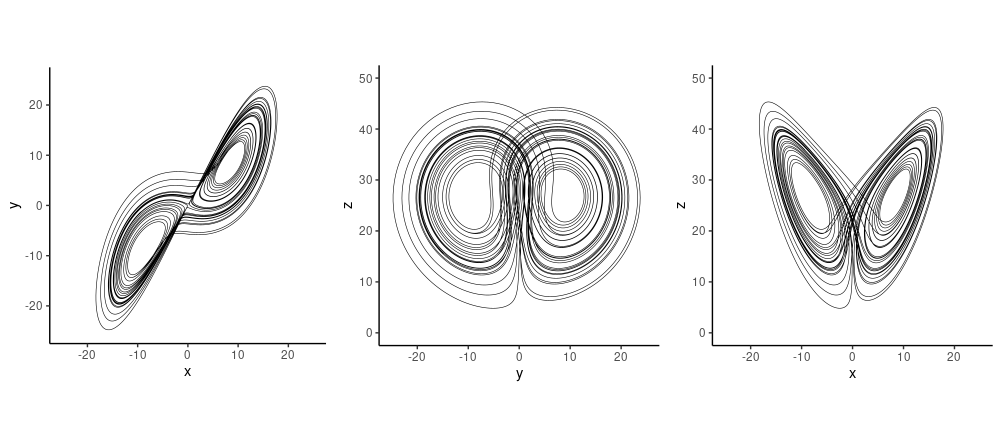Figure 6: Two-dimensional projections of the Lorenz attractor for sigma = 10, rho = 28, beta = 8 / 3. On the right: the butterfly.

The butterfly is an attractor (as are the other two projections), but it is neither a point nor a cycle. It is an attractor in the sense that starting from a variety of different initial values, we end up in some sub-region of the state space, and we don’t get to escape no more. This is easier to see when watching evolution over time, as in this animation: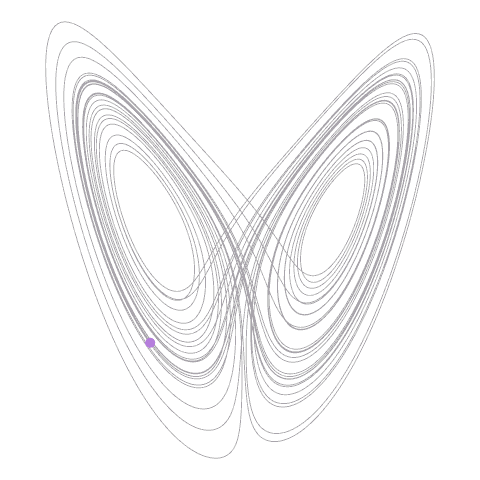Figure 7: How the Lorenz attractor traces out the famous “butterfly” shape.

Now, to plot the attractor in two dimensions, we threw away the third. But in “real life,” we don’t usually have too much information (although it may sometimes seem like we had). We might have a lot of measurements, but these don’t usually reflect the actual state variables we’re interested in. In these cases, we may want to actually add information.

### Embeddings (as a non-DL term), or: Undoing the projection

Assume that instead of all three variables of the Lorenz system, we had measured just one: $$x$$, the rate of convection. Often in nonlinear dynamics, the technique of delay coordinate embedding is used to enhance a series of univariate measurements.

In this method – or family of methods – the univariate series is augmented by time-shifted copies of itself. There are two decisions to be made: How many copies to add, and how big the delay should be. To illustrate, if we had a scalar series,

1 2 3 4 5 6 7 8 9 10 11 ...

a three-dimensional embedding with time delay 2 would look like this:

1 3 5
2 4 6
3 5 7
4 6 8
5 7 9
6 8 10
7 9 11
...

Of the two decisions to be made – number of shifted series and time lag – the first is a decision on the dimensionality of the reconstruction space. Various theorems, such as Taken's theorem, indicate bounds on the number of dimensions required, provided the dimensionality of the true state space is known – which, in real-world applications, often is not the case.The second has been of little interest to mathematicians, but is important in practice. In fact, Kantz and Schreiber argue that in practice, it is the product of both parameters that matters, as it indicates the time span represented by an embedding vector.

How are these parameters chosen? Regarding reconstruction dimensionality, the reasoning goes that even in chaotic systems, points that are close in state space at time $$t$$ should still be close at time $$t + \Delta t$$, provided $$\Delta t$$ is very small. So say we have two points that are close, by some metric, when represented in two-dimensional space. But in three dimensions, that is, if we don’t “project away” the third dimension, they are a lot more distant. As illustrated in :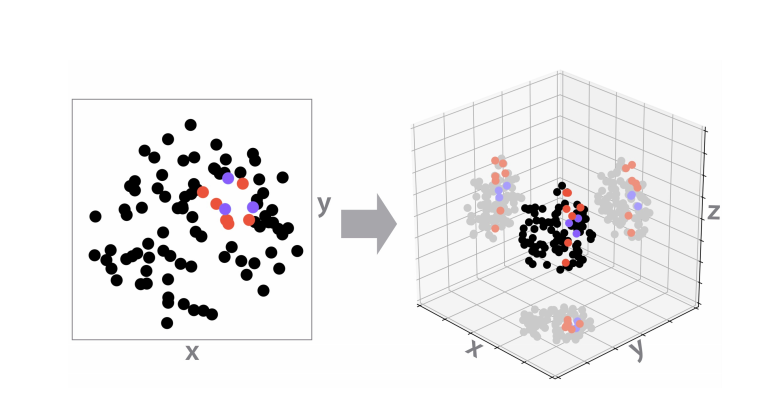Figure 8: In the two-dimensional projection on axes x and y, the red points are close neighbors. In 3d, however, they are separate. Compare with the blue points, which stay close even in higher-dimensional space. Figure from Gilpin (2020).

If this happens, then projecting down has eliminated some essential information. In 2d, the points were false neighbors. The false nearest neighbors (FNN) statistic can be used to determine an adequate embedding size, like this:

For each point, take its closest neighbor in $$m$$ dimensions, and compute the ratio of their distances in $$m$$ and $$m+1$$ dimensions. If the ratio is larger than some threshold $$t$$, the neighbor was false. Sum the number of false neighbors over all points. Do this for different $$m$$ and $$t$$, and inspect the resulting curves.

At this point, let’s look ahead at the autoencoder approach. The autoencoder will use that same FNN statistic as a regularizer, in addition to the usual autoencoder reconstruction loss. This will result in a new heuristic regarding embedding dimensionality that involves fewer decisions.

Going back to the classic method for an instant, the second parameter, the time lag, is even more difficult to sort out . Usually, mutual information is plotted for different delays and then, the first delay where it falls below some threshold is chosen. We don’t further elaborate on this question as it is rendered obsolete in the neural network approach. Which we’ll see now.

## Learning the Lorenz attractor

Our code closely follows the architecture, parameter settings, and data setup used in the reference implementation William provided. The loss function, especially, has been ported one-to-one.

The general idea is the following. An autoencoder – for example, an LSTM autoencoder as presented here – is used to compress the univariate time series into a latent representation of some dimensionality, which will constitute an upper bound on the dimensionality of the learned attractor. In addition to mean squared error between input and reconstructions, there will be a second loss term, applying the FNN regularizer. This results in the latent units being roughly ordered by importance, as measured by their variance. It is expected that somewhere in the listing of variances, a sharp drop will appear. The units before the drop are then assumed to encode the attractor of the system in question.

In this setup, there is still a choice to be made: how to weight the FNN loss. One would run training for different weights $$\lambda$$ and look for the drop. Surely, this could in principle be automated, but given the newness of the method – the paper was published this year – it makes sense to focus on thorough analysis first.

### Data generation

We use the deSolve package to generate data from the Lorenz equations.

library(deSolve)
library(tidyverse)

parameters <- c(sigma = 10,
rho = 28,
beta = 8/3)

initial_state <-
c(x = -8.60632853,
y = -14.85273055,
z = 15.53352487)

lorenz <- function(t, state, parameters) {
with(as.list(c(state, parameters)), {
dx <- sigma * (y - x)
dy <- x * (rho - z) - y
dz <- x * y - beta * z

list(c(dx, dy, dz))
})
}

times <- seq(0, 500, length.out = 125000)

lorenz_ts <-
ode(
y = initial_state,
times = times,
func = lorenz,
parms = parameters,
method = "lsoda"
) %>% as_tibble()

lorenz_ts[1:10,]
# A tibble: 10 x 4
time      x     y     z
<dbl>  <dbl> <dbl> <dbl>
1 0        -8.61 -14.9  15.5
2 0.00400  -8.86 -15.2  15.9
3 0.00800  -9.12 -15.6  16.3
4 0.0120   -9.38 -16.0  16.7
5 0.0160   -9.64 -16.3  17.1
6 0.0200   -9.91 -16.7  17.6
7 0.0240  -10.2  -17.0  18.1
8 0.0280  -10.5  -17.3  18.6
9 0.0320  -10.7  -17.7  19.1
10 0.0360  -11.0  -18.0  19.7

We’ve already seen the attractor, or rather, its three two-dimensional projections, in figure 6 above. But now our scenario is different. We only have access to $$x$$, a univariate time series. As the time interval used to numerically integrate the differential equations was rather tiny, we just use every tenth observation.

obs <- lorenz_ts %>%
select(time, x) %>%
filter(row_number() %% 10 == 0)

ggplot(obs, aes(time, x)) +
geom_line() +
coord_cartesian(xlim = c(0, 100)) +
theme_classic()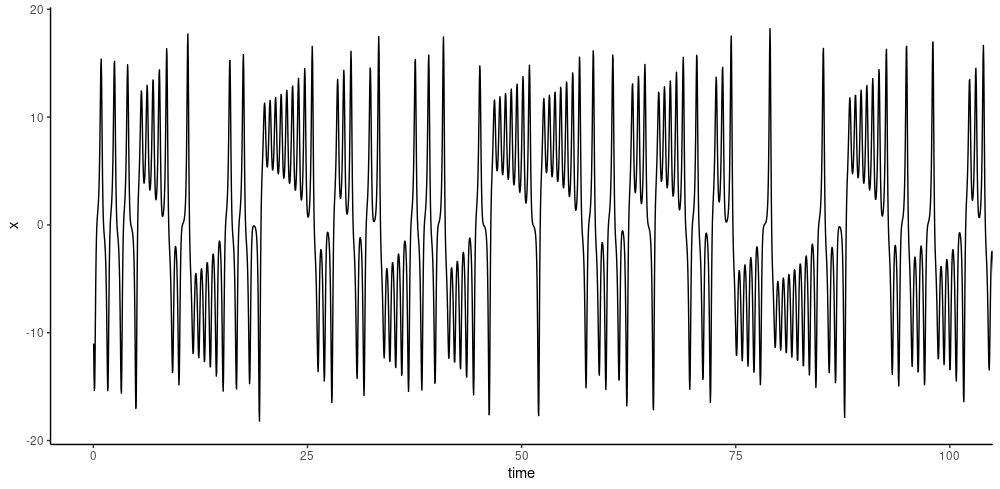Figure 9: Convection rates as a univariate time series.

### Preprocessing

The first half of the series is used for training. The data is scaled and transformed into the three-dimensional form expected by recurrent layers.

library(keras)
library(tfdatasets)
library(tfautograph)
library(reticulate)
library(purrr)

# scale observations
obs <- obs %>% mutate(
x = scale(x)
)

# generate timesteps
n <- nrow(obs)
n_timesteps <- 10

gen_timesteps <- function(x, n_timesteps) {
do.call(rbind,
purrr::map(seq_along(x),
function(i) {
start <- i
end <- i + n_timesteps - 1
out <- x[start:end]
out
})
) %>%
na.omit()
}

# train with start of time series, test with end of time series
x_train <- gen_timesteps(as.matrix(obs$x)[1:(n/2)], n_timesteps) x_test <- gen_timesteps(as.matrix(obs$x)[(n/2):n], n_timesteps)

# add required dimension for features (we have one)
dim(x_train) <- c(dim(x_train), 1)
dim(x_test) <- c(dim(x_test), 1)

# some batch size (value not crucial)
batch_size <- 100

# transform to datasets so we can use custom training
ds_train <- tensor_slices_dataset(x_train) %>%
dataset_batch(batch_size)

ds_test <- tensor_slices_dataset(x_test) %>%
dataset_batch(nrow(x_test))

### Autoencoder

With newer versions of TensorFlow (>= 2.0, certainly if >= 2.2), autoencoder-like models are best coded as custom models, and trained in an “autographed” loop.6

The encoder is centered around a single LSTM layer, whose size determines the maximum dimensionality of the attractor. The decoder then undoes the compression – again, mainly using a single LSTM.

# size of the latent code
n_latent <- 10L
n_features <- 1

encoder_model <- function(n_timesteps,
n_features,
n_latent,
name = NULL) {

keras_model_custom(name = name, function(self) {

self$noise <- layer_gaussian_noise(stddev = 0.5) self$lstm <-  layer_lstm(
units = n_latent,
input_shape = c(n_timesteps, n_features),
return_sequences = FALSE
)
self$batchnorm <- layer_batch_normalization() function (x, mask = NULL) { x %>% self$noise() %>%
self$lstm() %>% self$batchnorm()
}
})
}

decoder_model <- function(n_timesteps,
n_features,
n_latent,
name = NULL) {

keras_model_custom(name = name, function(self) {

self$repeat_vector <- layer_repeat_vector(n = n_timesteps) self$noise <- layer_gaussian_noise(stddev = 0.5)
self$lstm <- layer_lstm( units = n_latent, return_sequences = TRUE, go_backwards = TRUE ) self$batchnorm <- layer_batch_normalization()
self$elu <- layer_activation_elu() self$time_distributed <- time_distributed(layer = layer_dense(units = n_features))

function (x, mask = NULL) {
x %>%
self$repeat_vector() %>% self$noise() %>%
self$lstm() %>% self$batchnorm() %>%
self$elu() %>% self$time_distributed()
}
})
}

encoder <- encoder_model(n_timesteps, n_features, n_latent)
decoder <- decoder_model(n_timesteps, n_features, n_latent)

### Loss

As already explained above, the loss function we train with is twofold. On the one hand, we compare the original inputs with the decoder outputs (the reconstruction), using mean squared error:

mse_loss <- tf$keras$losses$MeanSquaredError( reduction = tf$keras$losses$Reduction$SUM) In addition, we try to keep the number of false neighbors small, by means of the following regularizer.7 loss_false_nn <- function(x) { # original values used in Kennel et al. (1992) rtol <- 10 atol <- 2 k_frac <- 0.01 k <- max(1, floor(k_frac * batch_size)) tri_mask <- tf$linalg$band_part( tf$ones(
shape = c(n_latent, n_latent),
dtype = tf$float32 ), num_lower = -1L, num_upper = 0L ) batch_masked <- tf$multiply(
tri_mask[, tf$newaxis,], x[tf$newaxis, reticulate::py_ellipsis()]
)

x_squared <- tf$reduce_sum( batch_masked * batch_masked, axis = 2L, keepdims = TRUE ) pdist_vector <- x_squared + tf$transpose(
x_squared, perm = c(0L, 2L, 1L)
) -
2 * tf$matmul( batch_masked, tf$transpose(batch_masked, perm = c(0L, 2L, 1L))
)

all_dists <- pdist_vector
all_ra <-
tf$sqrt((1 / ( batch_size * tf$range(1, 1 + n_latent, dtype = tf$float32) )) * tf$reduce_sum(tf$square( batch_masked - tf$reduce_mean(batch_masked, axis = 1L, keepdims = TRUE)
), axis = c(1L, 2L)))

all_dists <- tf$clip_by_value(all_dists, 1e-14, tf$reduce_max(all_dists))

top_k <- tf$math$top_k(-all_dists, tf$cast(k + 1, tf$int32))
top_indices <- top_k[]

neighbor_dists_d <- tf$gather(all_dists, top_indices, batch_dims = -1L) neighbor_new_dists <- tf$gather(
all_dists[2:-1, , ],
top_indices[1:-2, , ],
batch_dims = -1L
)

# Eq. 4 of Kennel et al. (1992)
scaled_dist <- tf$sqrt(( tf$square(neighbor_new_dists) -
tf$square(neighbor_dists_d[1:-2, , ])) / tf$square(neighbor_dists_d[1:-2, , ])
)

# Kennel condition #1
is_false_change <- (scaled_dist > rtol)
# Kennel condition #2
is_large_jump <-
(neighbor_new_dists > atol * all_ra[1:-2, tf$newaxis, tf$newaxis])

is_false_neighbor <-
tf$math$logical_or(is_false_change, is_large_jump)

total_false_neighbors <-
tf$cast(is_false_neighbor, tf$int32)[reticulate::py_ellipsis(), 2:(k + 2)]

reg_weights <- 1 -
tf$reduce_mean(tf$cast(total_false_neighbors, tf$float32), axis = c(1L, 2L)) reg_weights <- tf$pad(reg_weights, list(list(1L, 0L)))

activations_batch_averaged <-
tf$sqrt(tf$reduce_mean(tf$square(x), axis = 0L)) loss <- tf$reduce_sum(tf$multiply(reg_weights, activations_batch_averaged)) loss } MSE and FNN are added , with FNN loss weighted according to the essential hyperparameter of this model: fnn_weight <- 10 This value was experimentally chosen as the one best conforming to our look-for-the-highest-drop heuristic. ### Model training The training loop closely follows the aforementioned recipe on how to train with custom models and tfautograph. train_loss <- tf$keras$metrics$Mean(name='train_loss')
train_fnn <- tf$keras$metrics$Mean(name='train_fnn') train_mse <- tf$keras$metrics$Mean(name='train_mse')

train_step <- function(batch) {

with (tf$GradientTape(persistent = TRUE) %as% tape, { code <- encoder(batch) reconstructed <- decoder(code) l_mse <- mse_loss(batch, reconstructed) l_fnn <- loss_false_nn(code) loss <- l_mse + fnn_weight * l_fnn }) encoder_gradients <- tape$gradient(loss, encoder$trainable_variables) decoder_gradients <- tape$gradient(loss, decoder$trainable_variables) optimizer$apply_gradients(
purrr::transpose(list(encoder_gradients, encoder$trainable_variables)) ) optimizer$apply_gradients(
purrr::transpose(list(decoder_gradients, decoder$trainable_variables)) ) train_loss(loss) train_mse(l_mse) train_fnn(l_fnn) } training_loop <- tf_function(autograph(function(ds_train) { for (batch in ds_train) { train_step(batch) } tf$print("Loss: ", train_loss$result()) tf$print("MSE: ", train_mse$result()) tf$print("FNN loss: ", train_fnn$result()) train_loss$reset_states()
train_mse$reset_states() train_fnn$reset_states()

}))

for (epoch in 1:200) {
cat("Epoch: ", epoch, " -----------\n")
training_loop(ds_train)
}

After two hundred epochs, overall loss is at 2.67, with the MSE component at 1.8 and FNN at 0.09.

### Obtaining the attractor from the test set

We use the test set to inspect the latent code:

test_batch <- as_iterator(ds_test) %>% iter_next()
predicted <- encoder(test_batch) %>%
as.array(predicted) %>%
as_tibble()

predicted
# A tibble: 6,242 x 10
V1    V2         V3        V4        V5         V6        V7        V8       V9       V10
<dbl> <dbl>      <dbl>     <dbl>     <dbl>      <dbl>     <dbl>     <dbl>    <dbl>     <dbl>
1 0.439 0.401 -0.000614  -0.0258   -0.00176  -0.0000276  0.000276  0.00677  -0.0239   0.00906
2 0.415 0.504  0.0000481 -0.0279   -0.00435  -0.0000970  0.000921  0.00509  -0.0214   0.00921
3 0.389 0.619  0.000848  -0.0240   -0.00661  -0.000171   0.00106   0.00454  -0.0150   0.00794
4 0.363 0.729  0.00137   -0.0143   -0.00652  -0.000244   0.000523  0.00450  -0.00594  0.00476
5 0.335 0.809  0.00128   -0.000450 -0.00338  -0.000307  -0.000561  0.00407   0.00394 -0.000127
6 0.304 0.828  0.000631   0.0126    0.000889 -0.000351  -0.00167   0.00250   0.0115  -0.00487
7 0.274 0.769 -0.000202   0.0195    0.00403  -0.000367  -0.00220  -0.000308  0.0145  -0.00726
8 0.246 0.657 -0.000865   0.0196    0.00558  -0.000359  -0.00208  -0.00376   0.0134  -0.00709
9 0.224 0.535 -0.00121    0.0162    0.00608  -0.000335  -0.00169  -0.00697   0.0106  -0.00576
10 0.211 0.434 -0.00129    0.0129    0.00606  -0.000306  -0.00134  -0.00927   0.00820 -0.00447
# … with 6,232 more rows

As a result of the FNN regularizer, the latent code units should be ordered roughly by decreasing variance, with a sharp drop appearing some place (if the FNN weight has been chosen adequately).

For an fnn_weight of 10, we do see a drop after the first two units:

predicted %>% summarise_all(var)
# A tibble: 1 x 10
V1     V2      V3      V4      V5      V6      V7      V8      V9     V10
<dbl>  <dbl>   <dbl>   <dbl>   <dbl>   <dbl>   <dbl>   <dbl>   <dbl>   <dbl>
1 0.0739 0.0582 1.12e-6 3.13e-4 1.43e-5 1.52e-8 1.35e-6 1.86e-4 1.67e-4 4.39e-5

So the model indicates that the Lorenz attractor can be represented in two dimensions. If we nonetheless want to plot the complete (reconstructed) state space of three dimensions, we should reorder the remaining variables by magnitude of variance8. Here, this results in three projections of the set V1, V2 and V4: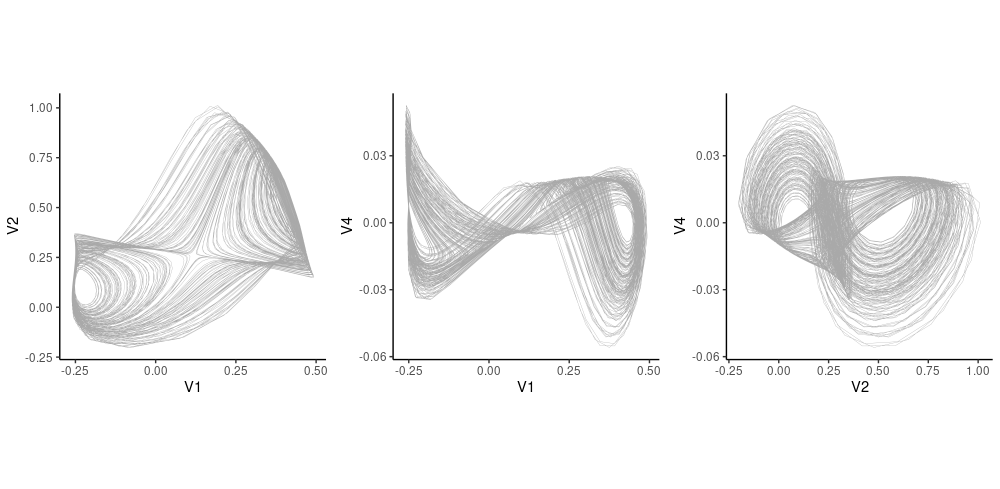Figure 10: Attractors as predicted from the latent code (test set). The three highest-variance variables were chosen.

## Wrapping up (for this time)

At this point, we’ve seen how to reconstruct the Lorenz attractor from data we did not train on (the test set), using an autoencoder regularized by a custom false nearest neighbors loss. It is important to stress that at no point was the network presented with the expected solution (attractor) – training was purely unsupervised.

This is a fascinating result. Of course, thinking practically, the next step is to obtain predictions on heldout data. Given how long this text has become already, we reserve that for a follow-up post. And again of course, we’re thinking about other datasets, especially ones where the true state space is not known beforehand. What about measurement noise? What about datasets that are not completely deterministic9? There is a lot to explore, stay tuned – and as always, thanks for reading!

Gilpin, William. 2020. “Deep Reconstruction of Strange Attractors from Time Series.” https://arxiv.org/abs/2002.05909.
Kantz, Holger, and Thomas Schreiber. 2004. Nonlinear Time Series Analysis. Cambridge University Press.
Kennel, Matthew B., Reggie Brown, and Henry D. I. Abarbanel. 1992. “Determining Embedding Dimension for Phase-Space Reconstruction Using a Geometrical Construction.” Phys. Rev. A 45 (March): 3403–11. https://doi.org/10.1103/PhysRevA.45.3403.
Sauer, Tim, James A. Yorke, and Martin Casdagli. 1991. Embedology.” Journal of Statistical Physics 65 (3-4): 579–616. https://doi.org/10.1007/BF01053745.
Strang, Gilbert. 2019. Linear Algebra and Learning from Data. Wellesley Cambridge Press.
Strogatz, Steven. 2015. Nonlinear Dynamics and Chaos: With Applications to Physics, Biology, Chemistry, and Engineering. Westview Press.

1. For many popular activation functions at least (such as ReLU). See e.g. .↩︎

2. The paper is also accompanied by a Python implementation.↩︎

3. To people who want to learn more about this topic, the usual recommendation is . Personally I prefer another source, which I can’t recommend highly enough: Santa Fe Institute’s Nonlinear Dynamics: Mathematical and Computational Approaches, taught by Liz Bradley.↩︎

4. See e.g. Wikipedia for some history and links to sources.↩︎

5. In discrete systems, like the logistic map, a single dimension is enough.↩︎

6. See the custom training tutorial for a blueprint.↩︎

7. See the appendix of for a pseudocode-like documentation.↩︎

8. As per author recommendation (personal communication).↩︎

9. See for detailed discussions on using methodology from nonlinear deterministic systems analysis for noisy and/or partly-stochastic data.

↩︎

### Reuse

Text and figures are licensed under Creative Commons Attribution CC BY 4.0. The figures that have been reused from other sources don't fall under this license and can be recognized by a note in their caption: "Figure from ...".

### Citation

Keydana (2020, June 24). Posit AI Blog: Deep attractors: Where deep learning meets chaos. Retrieved from https://blogs.rstudio.com/tensorflow/posts/2020-06-24-deep-attractors/
@misc{keydanadeepattractors,
}